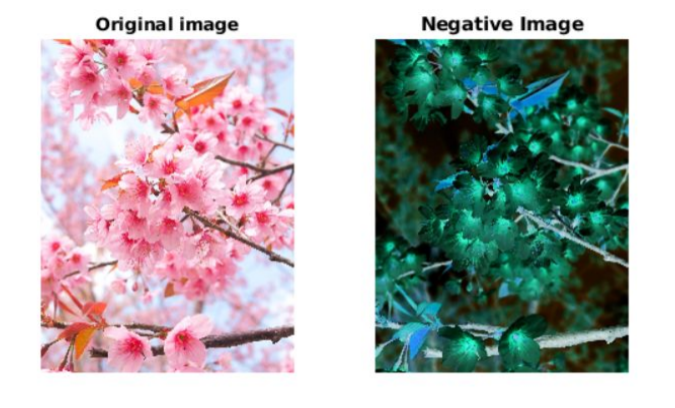# Negative of an image in MATLAB

The negative of an image is achieved by replacing the intensity ‘i’ in the original image by ‘i-1’, i.e. the darkest pixels will become the brightest and the brightest pixels will become the darkest. Image negative is produced by subtracting each pixel from the maximum intensity value.
For example in an 8-bit grayscale image, the max intensity value is 255, thus each pixel is subtracted from 255 to produce the output image.
The transformation function used in image negative is :

```s = T(r) = (L – 1) – r

Where L - 1 is the max intensity value,
s is the output pixel value and
r is the input pixel value
```

Algorithm

1. Read RGB color image into the MATLAB environment using Matlab inbuilt function `imread()`
2. Calculate the levels of the image, for example an 8-bit image has 256 levels
3. Use the formula stated above on every pixel of the image to get corresponding negative pixel value.
4. Convert each RGB pixel value at location (i, j) to its negative image values and assign it to the corresponding location (i, j) of another matrix
5. Display the negative image using Matlab in-built `imshow()` function.

 `% reading the RGB file into the Matlab environment ` `skI = imread(``"sakura.jpg"``);    ` `subplot(1, 2, 1), ` ` `  `% displaying the RGB image ` `imshow(skI); ` `title(``"Original image"``); ` ` `  `% levels of the 8-bit image ` `L = 2 ^ 8;     ` ` `  `% finding the negative                    ` `neg = (L - 1) - skI; ` `subplot(1, 2, 2), ` ` `  `% displaying the negative image ` `imshow(neg); ` `title(``"Negative Image"``) `

Output :Attention reader! Don’t stop learning now. Get hold of all the important CS Theory concepts for SDE interviews with the CS Theory Course at a student-friendly price and become industry ready.

My Personal Notes arrow_drop_upCheck out this Author's contributed articles.

If you like GeeksforGeeks and would like to contribute, you can also write an article using contribute.geeksforgeeks.org or mail your article to contribute@geeksforgeeks.org. See your article appearing on the GeeksforGeeks main page and help other Geeks.

Please Improve this article if you find anything incorrect by clicking on the "Improve Article" button below.

Article Tags :

Be the First to upvote.

Please write to us at contribute@geeksforgeeks.org to report any issue with the above content.